Function Repository Resource:

# TotalVariation

Get the total variation of a matrix

Contributed by: Wolfram Research
 ResourceFunction["TotalVariation"][matrix] gives the total variation for matrix.

## Details and Options

ResourceFunction["TotalVariation"][matrix] effectively gives the trace of the covariance matrix for matrix.
ResourceFunction["TotalVariation"][matrix] is equivalent to Total[Variance[matrix]].

## Examples

### Basic Examples (2)

TotalVariation of bivariate data:

 In:=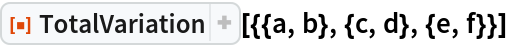Out=Assume all symbols are real and simplify:

 In:=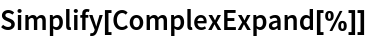Out=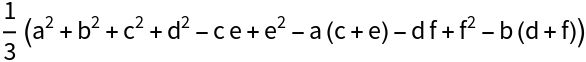### Properties & Relations (2)

TotalVariation is equivalent to the trace of the covariance matrix:

 In:=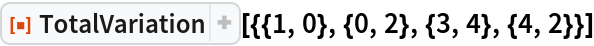Out=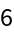In:=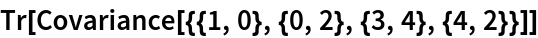Out=TotalVariation is equivalent to the sum of column variances:

 In:=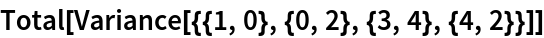Out=### Options (1)

Use the maximum likelihood estimation:

 In:=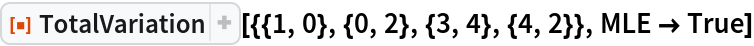Out=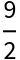## Requirements

Wolfram Language 11.3 (March 2018) or above

## Version History

• 1.0.0 – 06 February 2019